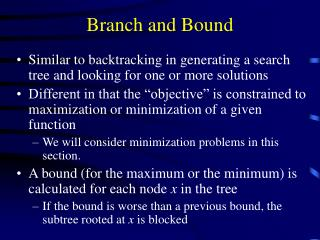# Branch and Bound - PowerPoint PPT PresentationDownload PresentationBranch and Bound

Branch and BoundDownload Presentation## Branch and Bound

- - - - - - - - - - - - - - - - - - - - - - - - - - - E N D - - - - - - - - - - - - - - - - - - - - - - - - - - -
##### Presentation Transcript

1. Branch and Bound • Similar to backtracking in generating a search tree and looking for one or more solutions • Different in that the “objective” is constrained to maximization or minimization of a given function • We will consider minimization problems in this section. • A bound (for the maximum or the minimum) is calculated for each node x in the tree • If the bound is worse than a previous bound, the subtree rooted at x is blocked

2. Branch and Bound • In order for Branch and Bound to work, the cost function Cost must satisfy the following condition on all partial solutions (x1, x2, ..., xk–1) and their extensions (x1, x2, ..., xk): Cost (x1, x2, ..., xk–1)  Cost(x1, x2, ..., xk) • Therefore, a partial solution can be discarded if its cost is greater than or equal to a previously computed solution

3. Branch and Bound: Example • Traveling Salesman Problem (TSP): Given a set of cities and a cost function that is defined on each pair of cities, find a tour of minimum cost • The cost function may be ................................... • An instance of TSP is given by a matrix of non-negative values • A lower bound y is associated such that the cost of any complete tour that visits cities x1, x2, ..., xk , in this order, must be at least y.

4. Branch and Bound: Example • Important Notes: • Each complete tour must contain exactly one edge and its associated cost from each row and each column of the cost matrix. • If a constant r is subtracted from every entry in a row or a column of the cost matrix, the cost of any tour under the new matrix is exactly r less than the cost of the same tour under the original matrix.

5. Branch and Bound: Example • Idea of the Solution: Reducethe cost matrix so that each row or column contains at least one entry that is equal to 0. Such a matrix is called a reduction of the original matrix. • In general, let (r1, r2, ..., rn) and (c1, c2, ..., cn) be the amounts subtracted from rows 1 to n and columns 1 to n, respectively, in an n  n cost matrix A. Then is a lower bound on the cost of any complete tour

6. Branch and Bound: Example • After creating the reduction matrix • The lower bound is computed • Initially, it is the value y of the reduction matrix • An edge (x, y) is chosen from the matrix • Two children of the current node are constructed where • The right node represents all solutions that exclude edge (x, y) • That is why the cost of (x, y) is set to  • The left node represents all solutions that include edge (x, y) • Row x and column y are removed from the cost matrix. Why? • Since all solutions use the edge (x, y), they will not use edge (y, x) and therefore the cost of (y, x) can be set to  • The above is also applied for the current “paths” • The lower bound in each child is computed by adding the current value of y into the current lower bound when computing the reduction matrix of each child. • Choose the subtree with minimum bound in order to apply branch and bound